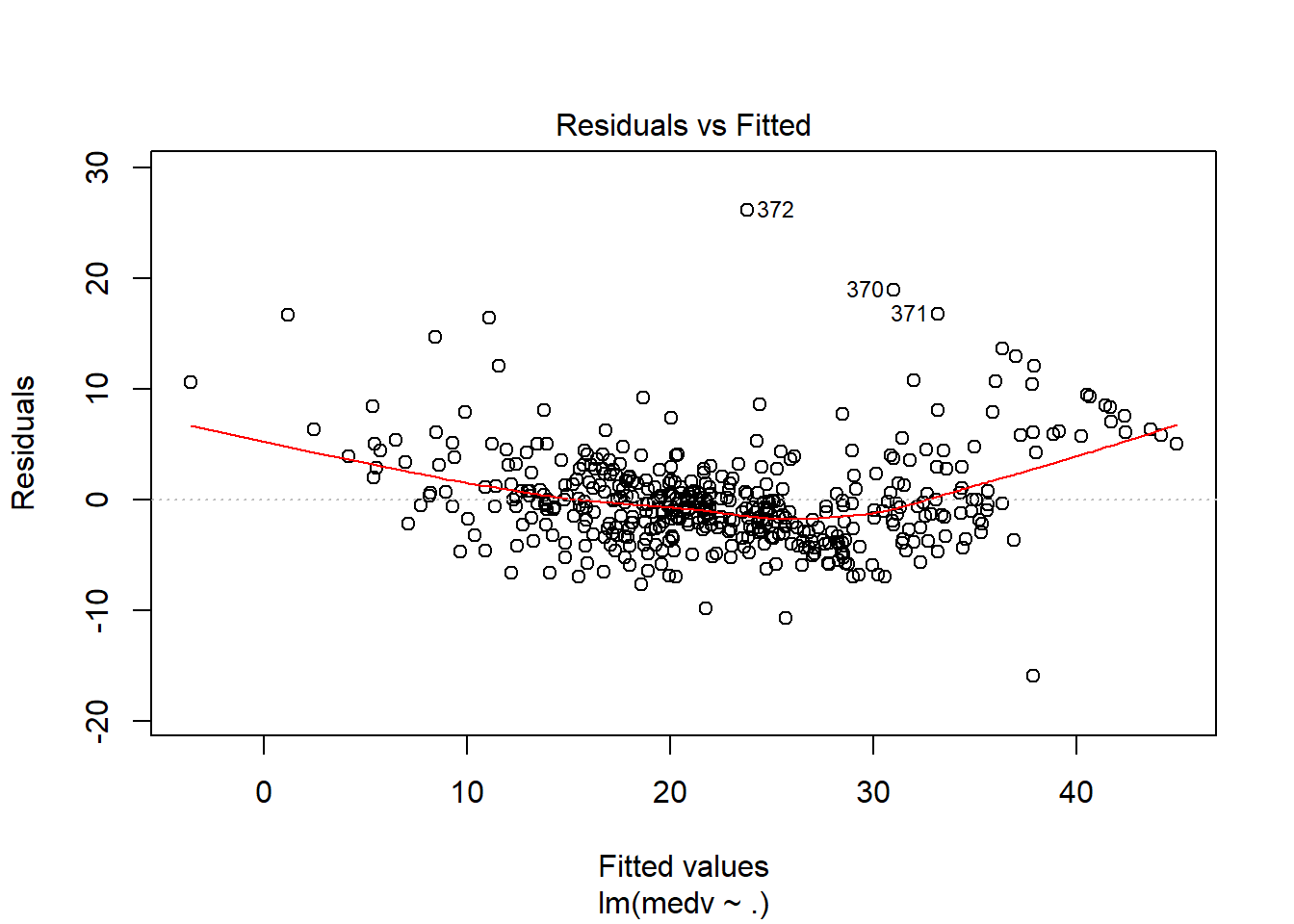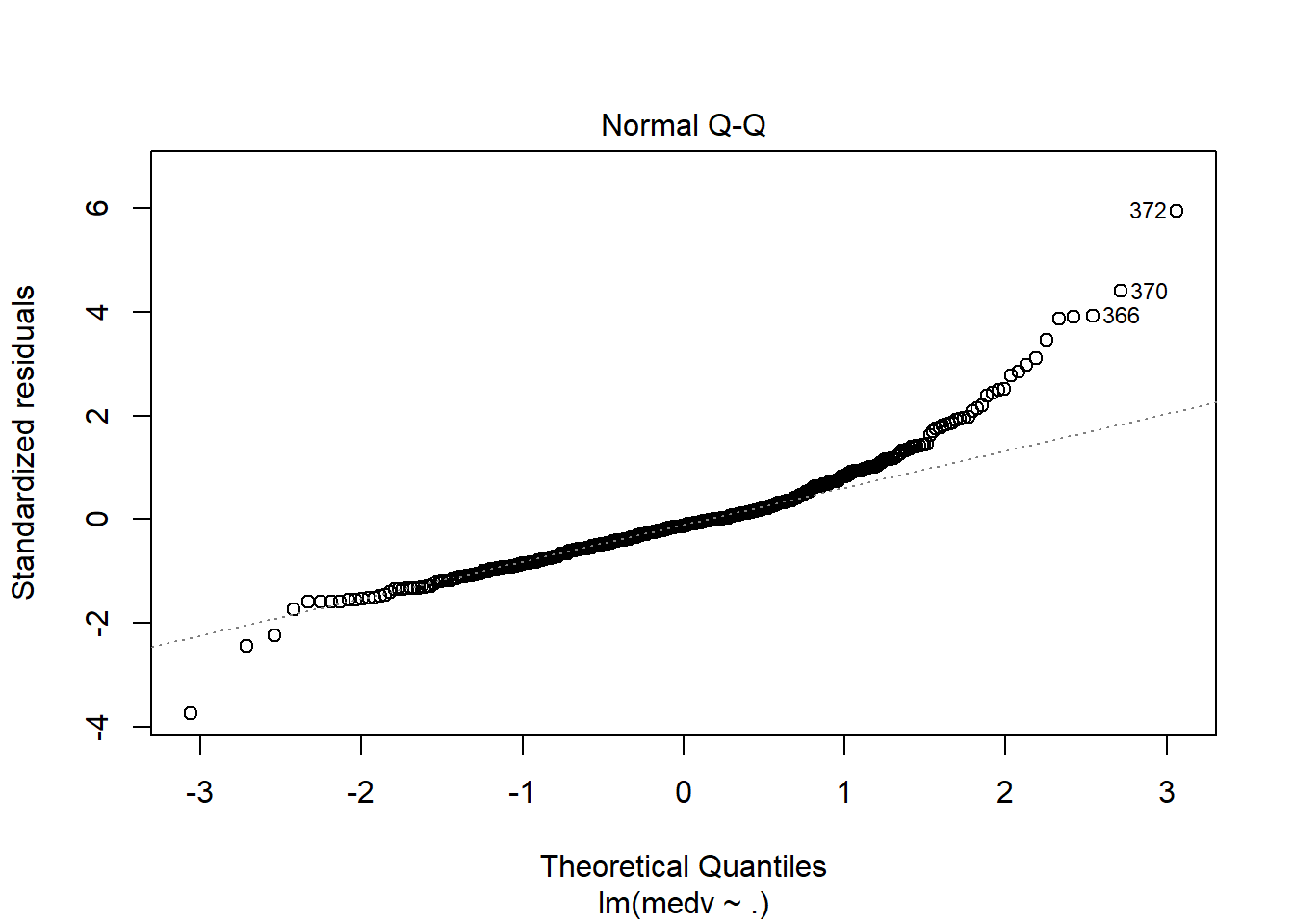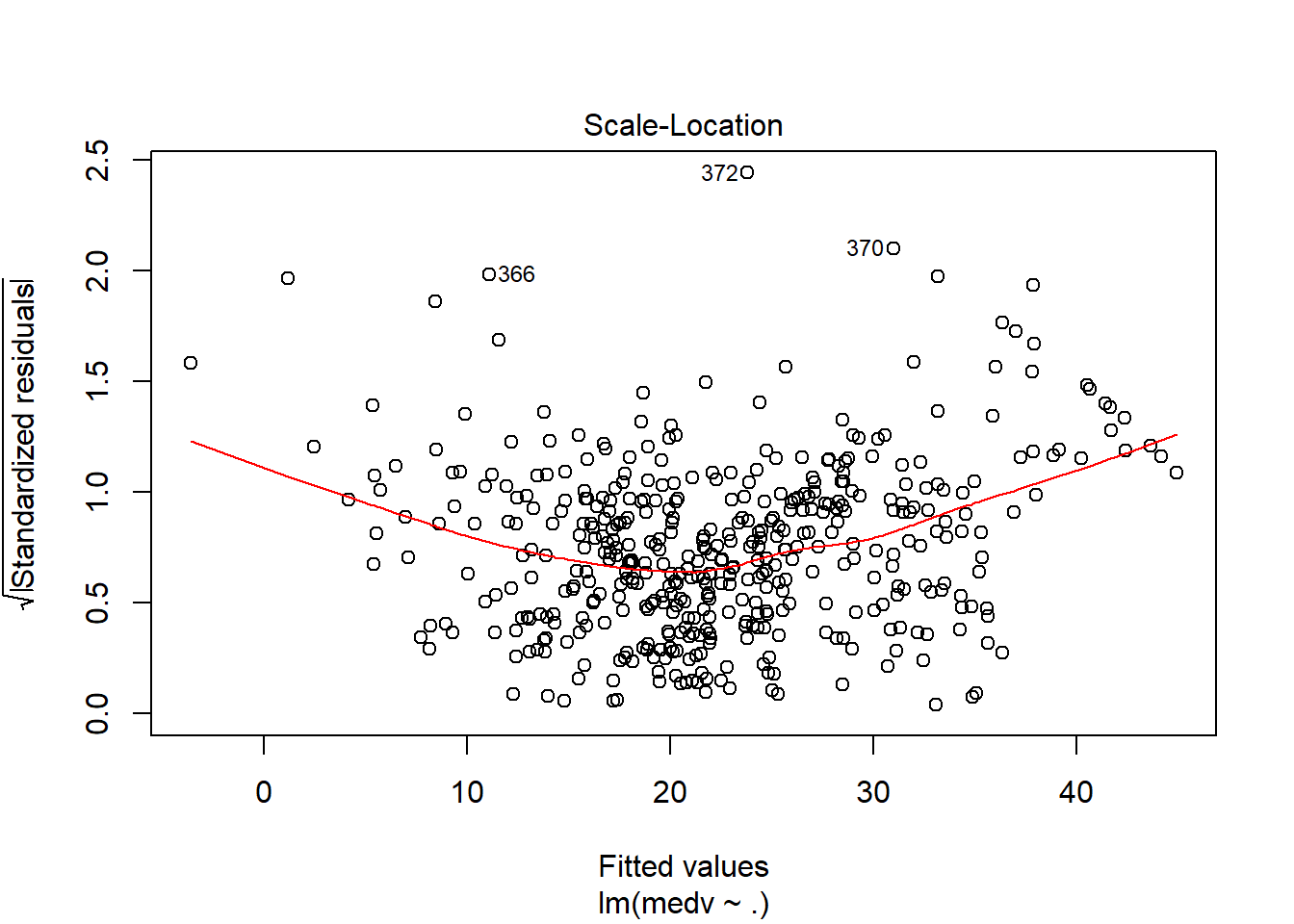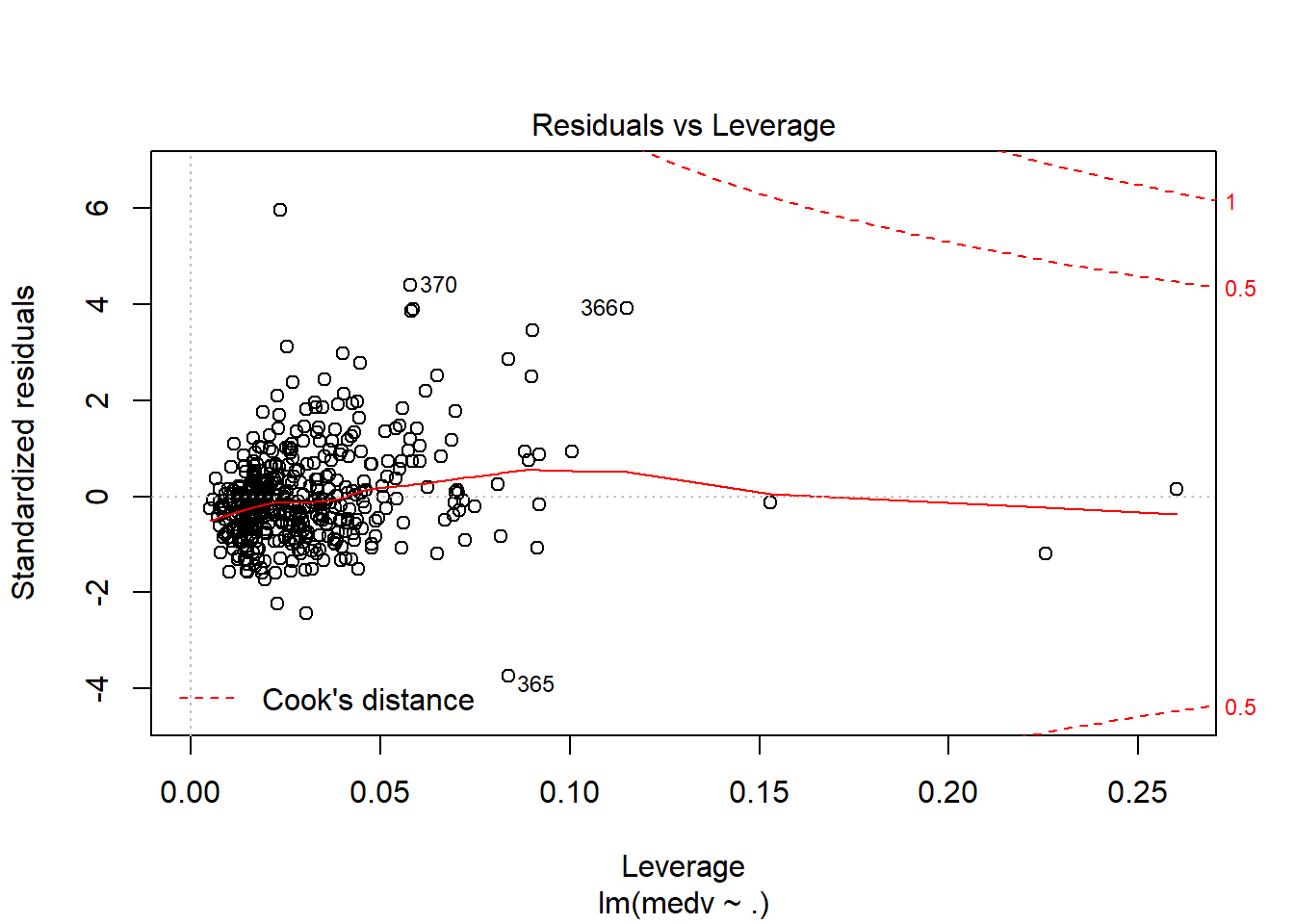# 1 Objective

The objective of this case is to get you started with regression model building, variable selection, and model evaluation in R.

We use Boston Housing Data as an illustrative example in this lab. We learn basic linear regression and analysis with R. Code in this file is not the only correct way to do things, however it is important for you to understand what each statement does. You will have to modify the code accordingly for your homework.

# 2 Boston Housing Data

Boston housing data is a built-in dataset in MASS package, so you do not need to download externally. Package MASS comes with R when you installed R, so no need to use install.packages(MASS) to download and install, but you do need to load this package.

library(MASS)
data(Boston); #this data is in MASS package
colnames(Boston) 
##   "crim"    "zn"      "indus"   "chas"    "nox"     "rm"      "age"
##   "dis"     "rad"     "tax"     "ptratio" "black"   "lstat"   "medv"

You can find details of the dataset from help document.

?Boston

The original data are 506 observations on 14 variables, medv being the response variable $$y$$:

## 2.2 EDA

We have introduced many EDA techniques in lab 2. We will briefly go through some of them here.

dim(Boston) 
##  506  14
names(Boston)
##   "crim"    "zn"      "indus"   "chas"    "nox"     "rm"      "age"
##   "dis"     "rad"     "tax"     "ptratio" "black"   "lstat"   "medv"
str(Boston)
## 'data.frame':    506 obs. of  14 variables:
##  $crim : num 0.00632 0.02731 0.02729 0.03237 0.06905 ... ##$ zn     : num  18 0 0 0 0 0 12.5 12.5 12.5 12.5 ...
##  $indus : num 2.31 7.07 7.07 2.18 2.18 2.18 7.87 7.87 7.87 7.87 ... ##$ chas   : int  0 0 0 0 0 0 0 0 0 0 ...
##  $nox : num 0.538 0.469 0.469 0.458 0.458 0.458 0.524 0.524 0.524 0.524 ... ##$ rm     : num  6.58 6.42 7.18 7 7.15 ...
##  $age : num 65.2 78.9 61.1 45.8 54.2 58.7 66.6 96.1 100 85.9 ... ##$ dis    : num  4.09 4.97 4.97 6.06 6.06 ...
##  $rad : int 1 2 2 3 3 3 5 5 5 5 ... ##$ tax    : num  296 242 242 222 222 222 311 311 311 311 ...
##  $ptratio: num 15.3 17.8 17.8 18.7 18.7 18.7 15.2 15.2 15.2 15.2 ... ##$ black  : num  397 397 393 395 397 ...
##  $lstat : num 4.98 9.14 4.03 2.94 5.33 ... ##$ medv   : num  24 21.6 34.7 33.4 36.2 28.7 22.9 27.1 16.5 18.9 ...
summary(Boston)
##       crim                zn             indus            chas
##  Min.   : 0.00632   Min.   :  0.00   Min.   : 0.46   Min.   :0.00000
##  1st Qu.: 0.08204   1st Qu.:  0.00   1st Qu.: 5.19   1st Qu.:0.00000
##  Median : 0.25651   Median :  0.00   Median : 9.69   Median :0.00000
##  Mean   : 3.61352   Mean   : 11.36   Mean   :11.14   Mean   :0.06917
##  3rd Qu.: 3.67708   3rd Qu.: 12.50   3rd Qu.:18.10   3rd Qu.:0.00000
##  Max.   :88.97620   Max.   :100.00   Max.   :27.74   Max.   :1.00000
##       nox               rm             age              dis
##  Min.   :0.3850   Min.   :3.561   Min.   :  2.90   Min.   : 1.130
##  1st Qu.:0.4490   1st Qu.:5.886   1st Qu.: 45.02   1st Qu.: 2.100
##  Median :0.5380   Median :6.208   Median : 77.50   Median : 3.207
##  Mean   :0.5547   Mean   :6.285   Mean   : 68.57   Mean   : 3.795
##  3rd Qu.:0.6240   3rd Qu.:6.623   3rd Qu.: 94.08   3rd Qu.: 5.188
##  Max.   :0.8710   Max.   :8.780   Max.   :100.00   Max.   :12.127
##  Min.   : 1.000   Min.   :187.0   Min.   :12.60   Min.   :  0.32
##  1st Qu.: 4.000   1st Qu.:279.0   1st Qu.:17.40   1st Qu.:375.38
##  Median : 5.000   Median :330.0   Median :19.05   Median :391.44
##  Mean   : 9.549   Mean   :408.2   Mean   :18.46   Mean   :356.67
##  3rd Qu.:24.000   3rd Qu.:666.0   3rd Qu.:20.20   3rd Qu.:396.23
##  Max.   :24.000   Max.   :711.0   Max.   :22.00   Max.   :396.90
##      lstat            medv
##  Min.   : 1.73   Min.   : 5.00
##  1st Qu.: 6.95   1st Qu.:17.02
##  Median :11.36   Median :21.20
##  Mean   :12.65   Mean   :22.53
##  3rd Qu.:16.95   3rd Qu.:25.00
##  Max.   :37.97   Max.   :50.00

We skip the Exploratory Data Analysis (EDA) in this notes, but you should not omit it in your HW and Cases. EDA is very important and always the first analysis to do before any modeling.

## 2.3 Preparation

### 2.3.1 Splitting data to training and testing samples

Next we sample 90% of the original data and use it as the training set. The remaining 10% is used as test set. The regression model will be built on the training set and future performance of your model will be evaluated with the test set.

sample_index <- sample(nrow(Boston),nrow(Boston)*0.90)
Boston_train <- Boston[sample_index,]
Boston_test <- Boston[-sample_index,]

### 2.3.2 (Optional) Standardization

If we want our results to be invariant to the units and the parameter estimates $$\beta_i$$ to be comparible, we can standardize the variables. Essentially we are replacing the original values with their z-score.

1st Way: create new variables manually.

Boston$sd.crim <- (Boston$crim-mean(Boston$crim))/sd(Boston$crim); 

This does the same thing.

Boston$sd.crim <- scale(Boston$crim); 

2nd way: If you have a lot of variables to standardize then the above is not very pleasing to do. You can use a loop like this. It standardizes every varables other than the last one which is $$y$$.

for (i in 1:(ncol(Boston_train)-1)){
Boston_train[,i] <- scale(Boston_train[,i])
}

The technique is not as important in linear regression because it will only affect the interpretation but not the model estimation and inference.

go to top

# 3 Model Building

You task is to build a best model with training data. You can refer to the regression and variable selection code on the slides for more detailed description of linear regression.

The following model includes all $$x$$ varables in the model

model_1 <- lm(medv~crim+zn+chas+nox+rm+dis+rad+tax+ptratio+black+lstat, data=Boston_train)

To include all variables in the model, you can write the statement this simpler way.

model_1 <- lm(medv~., data=Boston_train)
summary(model_1)
##
## Call:
## lm(formula = medv ~ ., data = Boston_train)
##
## Residuals:
##      Min       1Q   Median       3Q      Max
## -15.9532  -2.5929  -0.5149   1.6367  26.1959
##
## Coefficients:
##             Estimate Std. Error t value Pr(>|t|)
## (Intercept)  22.5066     0.2088 107.787  < 2e-16 ***
## crim         -0.5675     0.2975  -1.908 0.057087 .
## zn            0.9335     0.3156   2.958 0.003258 **
## indus         0.2041     0.4148   0.492 0.622917
## chas          0.5651     0.2174   2.600 0.009645 **
## nox          -1.8859     0.4426  -4.261 2.49e-05 ***
## rm            3.2063     0.2933  10.931  < 2e-16 ***
## age          -0.1905     0.3685  -0.517 0.605470
## dis          -2.6338     0.4090  -6.440 3.14e-10 ***
## rad           2.0130     0.5942   3.388 0.000767 ***
## tax          -1.7277     0.6455  -2.677 0.007713 **
## ptratio      -2.0819     0.2807  -7.418 6.16e-13 ***
## black         0.8161     0.2472   3.301 0.001043 **
## lstat        -3.3268     0.3612  -9.211  < 2e-16 ***
## ---
## Signif. codes:  0 '***' 0.001 '**' 0.01 '*' 0.05 '.' 0.1 ' ' 1
##
## Residual standard error: 4.454 on 441 degrees of freedom
## Multiple R-squared:  0.7692, Adjusted R-squared:  0.7624
## F-statistic: 113.1 on 13 and 441 DF,  p-value: < 2.2e-16

But, is this the model you want to use?

## 3.1 (Optional) Interaction terms in model

If you suspect the effect of one predictor x1 on the response y depends on the value of another predictor x2, you can add interaction terms in model. To specify interaction in the model, you put : between two variables with interaction effect. For example

lm(medv~crim+zn+crim:zn, data=Boston_train)
#The following way automatically add the main effects of crim and zn
lm(medv~crim*zn, data=Boston_train)

For now we will not investigate the interactions of variables.

# 4 Diagnostic Plots

The diagnostic plots are not as important when regression is used in predictive (supervised) data mining as when it is used in economics. However it is still good to know:

1. What the diagnostic plots should look like when no assumption is violated?

2. If there is something wrong, what assumptions are possibly violated?

3. What implications does it have on the analysis?

4. (How) can I fix it?

Roughly speaking, the table summarizes what you should look for in the following plots

Plot Name Good
Residual vs. Fitted No pattern, scattered around 0 line
Normal Q-Q Dots fall on dashed line
Residual vs. Leverage No observation with large Cook’s distance
plot(model_1)go to top

# 5 Model Assessment

Suppose that everything in model diagnostics is okay. In other words, the model we have built is fairly a valid model. Then we need to evaluate the model performance in terms of different metrics.

Commonly used metrics include MSE, (adjusted) $$R^2$$, AIC, BIC for in-sample performance, and MSPE for out-of-sample performance.

## 5.1 In-sample model evaluation (train error)

MSE of the regression, which is the square of ‘Residual standard error’ in the above summary. It is the sum of squared residuals(SSE) divided by degrees of freedom (n-p-1). In some textbooks the sum of squred residuals(SSE) is called residual sum of squares(RSS). MSE of the regression should be the unbiased estimator for variance of $$\epsilon$$, the error term in the regression model.

model_summary <- summary(model_1)
(model_summary$sigma)^2 ##  19.83811 $$R^2$$ of the model model_summary$r.squared
##  0.7692106

Adjusted-$$R^2$$ of the model, if you add a variable (or several in a group), SSE will decrease, $$R^2$$ will increase, but Adjusted-$$R^2$$ could go either way.

model_summary$adj.r.squared ##  0.7624072 AIC and BIC of the model, these are information criteria. Smaller values indicate better fit. AIC(model_1) ##  2666.374 BIC(model_1) ##  2728.179 BIC, AIC, and Adjusted $$R^2$$ have complexity penalty in the definition, thus when comparing across different models they are better indicators on how well the model will perform on future data. ## 5.2 Out-of-sample prediction (test error) To evaluate how the model performs on future data, we use predict() to get the predicted values from the test set. #pi is a vector that contains predicted values for test set. pi <- predict(object = model_1, newdata = Boston_test) Just as any other function, you can write the above statement the following way as long as the arguments are in the right order. pi <- predict(model_1, Boston_test) The most common measure is the Mean Squared Error (MSE): average of the squared differences between the predicted and actual values mean((pi - Boston_test$medv)^2)
##  25.75884

A less popular measure is the Mean Absolute Error (MAE). You can probably guess that here instead of taking the average of squared error, MAE is the average of absolute value of error.

mean(abs(pi - Boston_test$medv)) ##  3.149258 Note that if you ignore the second argument of predict(), it gives you the in-sample prediction on the training set: predict(model_1) Which is the same as model_1$fitted.values

go to top